Solution of Dirac equation for Eckart potential and trigonometric Manning Rosen potential using asymptotic iteration method
Arum Sari Resita , Suparmi A , Cari C
Physics Department, Sebelas Maret University, Jl. Ir. Sutami 36A Kentingan Surakarta 57126, Indonesia

Project supported by the Higher Education Project (Grant No. 698/UN27.11/PN/2015).

Abstract
Abstract

The Dirac equation for Eckart potential and trigonometric Manning Rosen potential with exact spin symmetry is obtained using an asymptotic iteration method. The combination of the two potentials is substituted into the Dirac equation, then the variables are separated into radial and angular parts. The Dirac equation is solved by using an asymptotic iteration method that can reduce the second order differential equation into a differential equation with substitution variables of hypergeometry type. The relativistic energy is calculated using Matlab 2011. This study is limited to the case of spin symmetry. With the asymptotic iteration method, the energy spectra of the relativistic equations and equations of orbital quantum number l can be obtained, where both are interrelated between quantum numbers. The energy spectrum is also numerically solved using the Matlab software, where the increase in the radial quantum number n r causes the energy to decrease. The radial part and the angular part of the wave function are defined as hypergeometry functions and visualized with Matlab 2011. The results show that the disturbance of a combination of the Eckart potential and trigonometric Manning Rosen potential can change the radial part and the angular part of the wave function.

1. Introduction

In 1928 Dirac investigated the relativistic wave equation covariance of the Schrodinger equation and proposed a matrix α , β , and relativistic energy to the first order I . [ 1 ] The Dirac equation describes the motion of a relativistic particle with spin 1/2, which is widely used to solve the problems of nuclear physics and high energy physics. [ 2 ] One of the important tasks of relativistic quantum mechanics is to find an accurate and exact solution of the Dirac equation for some noncentral physical potentials, which are a mixture of attractive scalar potential S ( r ) and repulsive vector potential V ( r ). Spin symmetry occurs when S ( r ) = V ( r ) and pseudospin (p-spin) symmetry occurs when S ( r ) = − V ( r ). The Dirac equation has been intensively investigated since it has important applications in quantum chemistry and nuclear physics. The Dirac equation is used to describe the motion of particles governed by a strong force when the relativistic effects are taken into account. [ 3 ]

The Dirac equation for the case of exact spin symmetric occurs when the difference in the magnitude betwen the repulsive vector potential and the attractive scalar potential is zero and the sum of the magnitudes of the repulsive vector potential and attractive scalar potential is equal to a given potential. The exact pseudo-spin symmetry occurs when the sum of the magnitude of the repulsive vector potential and the attractive scalar potential is zero and the difference between the vector potential and scalar potential is equal to a given potential, which is central or non-central. [ 4 ]

Solutions of the Dirac equation for some typical potentials under special cases of spin symmetry and pseudospin symmetry have been investigated. For spin symmetry κ ( κ + 1) = l ( l + 1) that gives κ = l = j + 1/2 for κ > 0 and κ = −( l + 1) = −( j + 1/2) for κ < 0, where κ is the eigenvalue of the spin orbit coupling operator, l is orbital quantum number, and j is the total angular momentum quantum number. For the pseudospin symmetry case, κ ( κ − 1) = ( + 1) that gives κ = + 1 = j + 1/2 for κ > 0 and κ = − = −( j + 1/2) for κ < 0, where is the quantum number of the pseudospin orbital. In nuclear physics, spin symmetry and pseudospin symmetry concepts have been used to study the aspect of deformed and super deformation nuclei. The concept of spin symmetry has been applied to the spectra of meson and antinucleon. [ 5 ]

Some researchers have investigated the Dirac equation by using a variety of potentials and different methods, such as the spin symmetry in the antinucleon spectrum and tensortype Coulomb potential with spin–orbit number κ in a state of spin symmetry and p-spin symmetry, [ 6 ] bound states of the Dirac equation with position-dependent mass for the Eckart potential, [ 7 ] the exact solution of Klein–Gordon with the Pöschl–Teller double-ring-shaped Coulomb potential, [ 8 ] the exact solution of the Dirac equation for the Coulomb potential plus NAD potential by using the Nikorov–Uvarov method, [ 9 ] the potential Deng–Fan and the Coulomb potential tensor using the asymptotic iteration method (AIM), [ 10 ] the potential Poschl–Teller plus the Manning Rosen radial section with the hypergeometry method, [ 11 ] the solution of Klein–Gordon equation for Hulthen non-central potential in radial part with Romanovski polynomial [ 12 ] and the solution of the Schrodinger equation with the Hulthen plus Manning– Rosen potential, [ 13 ] the Scarf potential with the new tensor coupling potential for spin and pseudospin symmetries using Romanovski polynomials, [ 14 ] for the q-deformed hyperbolic Poschl–Teller potential and the trigonometric Scarf II noncentral potential by using AIM, [ 15 ] eigensolutions of the deformed Woods–Saxon potential via AIM, [ 16 ] approximate solutions of the Klein Gordon equation with an improved Manning Rosen potential in D -dimensions using SUSYQM, [ 17 ] and eigen spectra of the Dirac equation for a deformed Woods–Saxon potential via the similarity transformation. [ 18 ]

In this study, we will solve the Dirac equation for the Eckart potential plus the trigonometric Manning Rosen potential in a state of spin symmetry using an asymptotic iteration method. The asymptotic iteration method is a method of solving the second-order differential equations. [ 20 ]

The rest of this paper is organized as follows. The asymptotic iteration method will be briefly reviewed in Section 2. In Section 3, we review the Eckart potential and trigonometric Manning Rosen potential, give a brief introduction to the Dirac equation with equal scalar and vector potentials, and apply the separation of variables in spherical coordinates. In Section 4, we solve the radial and angular parts of the Dirac equation by using the modified Eckart potential combined with trigonometric Manning Rosen potential and obtain the relativistic energy spectrum and wavefunction via the asymptotic iteration method. In Section 5, we present graphically some wavefunctions of the Dirac equation, present several relativistic energy spectra, and discuss some consequences of the results obtained. Finally, some conclusions are drawn from the present study in Section 6.

2. Asymptotic iteration method

This method is used to solve differential equation in the following form: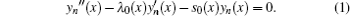Equation ( 1 ) can be solved by using iterations of λ k and s k ,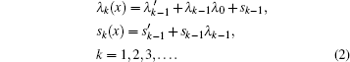Eigen values can be obtained using the following equation: [ 19 ]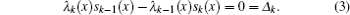While eigen functions of Eq. ( 1 ) can be obtained using [ 19 ]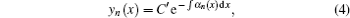where α can be solved by applying the asymptotic iteration k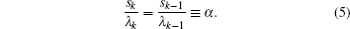Rewriting the second-order differential of Eq. ( 1 ) into a general form as follows: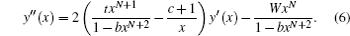The general formula for the exact solution of y n ( x ) is given by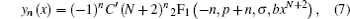where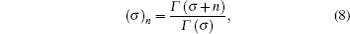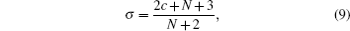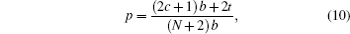C′ is the normalized constant, and 2 F 1 is the hipergeometry function. [ 20 ]

3. Dirac equation with equal scalar and vector Eckart potential combined with trigonometric Manning Rosen potential

The Dirac equation with scalar potential S ( r ) and vector potential V ( r ) (ℏ = 1, c = 1) is given by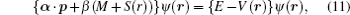where E is the relativistic energy, p is the momentum operator ( p = −i ), α and β are matrices in the following forms: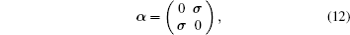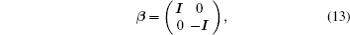where I is the 2 × 2 identity matrix and σ is the Pauli matrix, [ 14 ] whose components are expressed as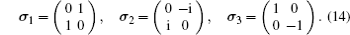In the Pauli–Dirac representation, let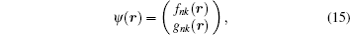we have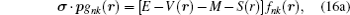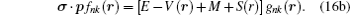For spin symmetry, equation ( 16a ) becomes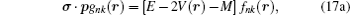where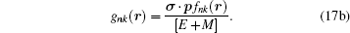Substituting Eq. ( 17b ) into Eq. ( 16b ) yields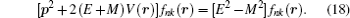In spherical coordinates, the Eckart potential combined with trigonometric Manning Rosen potential is defined as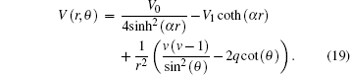Substitute Eq. ( 19 ) into Eq. ( 18 ) and simplify the resulting equation, and let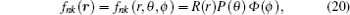then we will have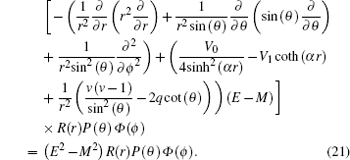Separating the variables in Eq. ( 21 ), we obtain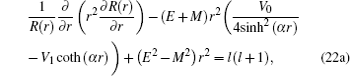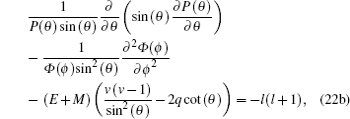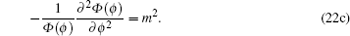Equation ( 22c ) is well known with its solution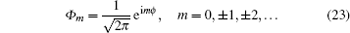The Dirac spin may be written according to the upper f ( r ) and lower g ( r ) components as follows: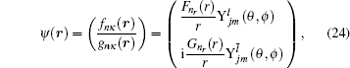where F n r ( r ) is the Dirac spin component, G n r ( r ) is the Dirac pseudospin component,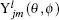and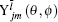are the spin and pseudospin spherical harmonics, l is the orbital quantum number, is the quantum number of the pseudo orbital, and m is the projection of the angular momentum on the z axis.

By substituting Eq. ( 24 ) to Eq. ( 11 ), we can immediately obtain two coupled ordinary differential equations for radial parts of Dirac eigen functions as follows: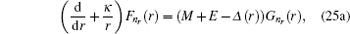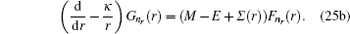Eliminating F n r ( r ) and G n r ( r ) in Eqs. ( 25a ) and ( 25b ), we obtain the second-order differential equation for F n r ( r ) and G n r ( r ) components of the Dirac wavefunction as follows: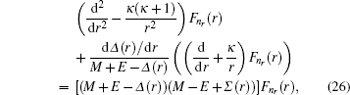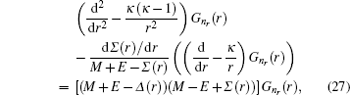where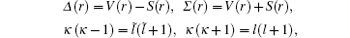κ is the quantum number of the spin orbital, l is the orbital quantum number, and the quantum number of the pseudospin orbital.

4. Analytical solution of radial and angular parts of the Dirac equation
4.1. Solution of the radial part

In the case of exact spin symmetry d Δ ( r )/d r = 0 and Δ ( r ) = C = const, equation ( 26 ) becomes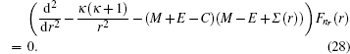We assume that Σ ( r ) is the Eckart potensial, which is defined as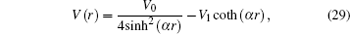and we use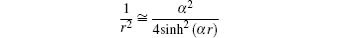as the centrifugal term. The radial Dirac equation takes the following form: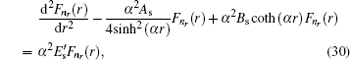where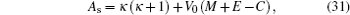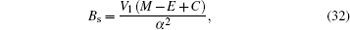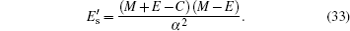By substituting variable coth ( αr ) = 1 − 2 z into Eq. ( 30 ), we have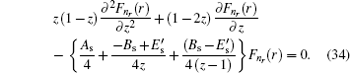By substituting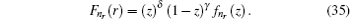into Eq. ( 34 ), we have the second-order differential equation in the following form: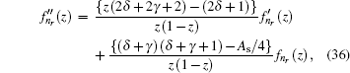where − B s + E s = 4 δ 2 and B s E s = 4 γ 2 .

By comparing this equation with Eq. ( 1 ), we can write λ 0 ( z ) and s 0 ( z ) values. By means of Eq. ( 2 ), we can calculate λ k ( z ) and s k ( z )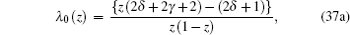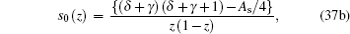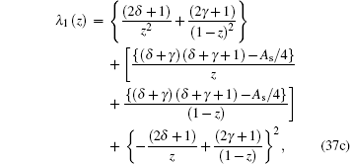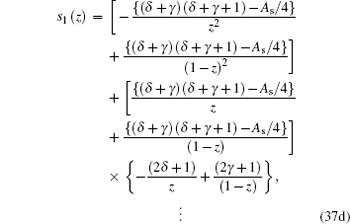Combining these results with the quantization condition given by Eq. ( 3 ) yields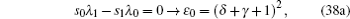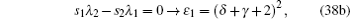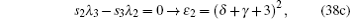where ɛ n r = A s /4.

When the above expressions are generalized into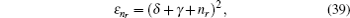the eigenvalues turn into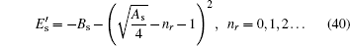By substituting Eqs. ( 31 )–( 33 ) into Eq. ( 40 ), we obtain energy eigenvalue E as follows: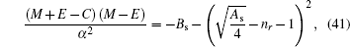where n r is the radial quantum number ( n r = 0,1,2…), l is orbital quantum number which is obtained from the angular part solution. The radial wavefunction can be obtained by using Eqs. ( 6 )–( 8 ), and Eq. ( 10 ), and we havesoFrom Eq. ( 6 ), we have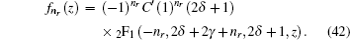By substituting Eq. ( 42 ) into Eq. ( 35 ), we have radial wavefunction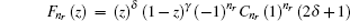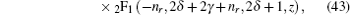where z = [1-coth ( αr )]/2, so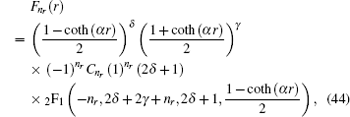where C n r is the radial normalization constant, 2 F 1 is the hypergeometry function, and (2 δ + 1) n r is a Pochamer symbol.

4.2. Solution of the angular part

For the angular part in Eq. ( 22b ), it can be obtained by using AIM to find the orbital quantum number. By multiplying Eq. ( 22b ) with P ( θ )/sin ( θ ), we have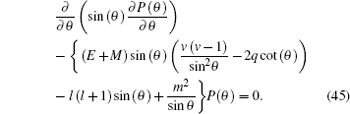Equation ( 45 ) must be simplified by using parameter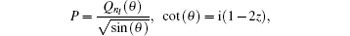and the simplified equation is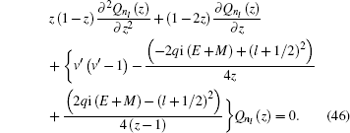By using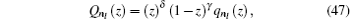and simplying it, equation ( 46 ) can be transformed to a hypergeometry differential equation: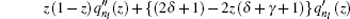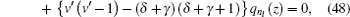where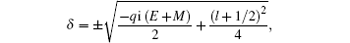and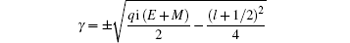with l being the orbital quantum number.

Equation ( 48 ) is the hypergeometry differential equation, so we must transform it into an AIM type by dividing Eq. ( 48 ) with z (1 − z ), then we have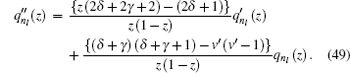From Eq. ( 49 ), we have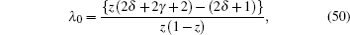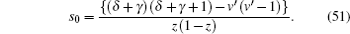To have this eigen value of the equation, further iterations λ k and s k , where k is the stated iteration, by using Eq. ( 3 ), the energy eigen value can be obtained, by using software Matlab,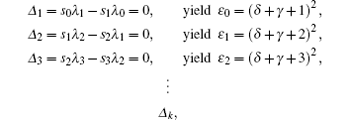where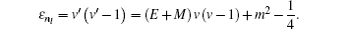When the above expressions are generalized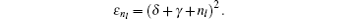Energy eigen value can be obtained by using the generalized Δ k , which yields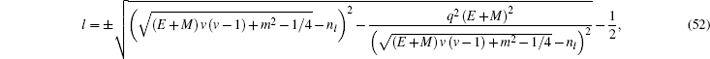where l is the orbital quantum number and n l is the angular quantum number.

Angular wavefunction can be obtained by using Eqs. ( 6 )–( 8 ), and ( 10 ), and we have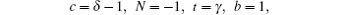soFrom Eq. ( 7 ), we have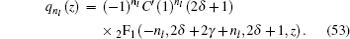Substituting Eq. ( 53 ) into Eq. ( 47 ) yields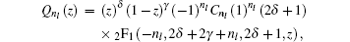where z = [1 + i cot ( θ )]/2, so the angular part wavefunction can be obtained as follows: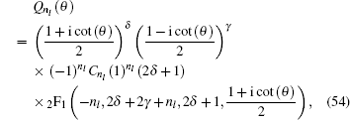with C n l being the angular normalization constant.

5. Result and discussion

In this section, we discuss several results obtained in the previous section. From the energy eigen value in Eq. ( 41 ) and the orbital quantum number in Eq. ( 52 ), by using Matlab, we have the numeric solution of the energy eigen value, and the results are listed in Table  1 with parameters v = 0.25, α = 0.5, V 0 = 0.4, V 1 = 0.6, and M = 5 fm −1 . The positive value is taken due to the spin symmetric limit. Table  1 shows that the increases of values q and n r in the same quantum state cause the energy eigenvalue to decrease.Table 1.

Energy eigenvalues corresponding to several states of a particle under the influence of the Eckart potential and trigonometric Manning Rosen potential.

.

Table  2 lists the energy eigen values with different values of potential constant V 0 and V 1 , which are plotted in Fig.  1 for different values of V 0 and V 1 . Figure  1 shows that the increases of potential constant V 0 and V 1 cause the energy to decrease.Table 2.

Energy eigenvalues (in fm −1 ) with M = 5; C = 5; v = 0.25; q = 0.2; α = 0.5, for a particle under the influences of the Eckart potential and the trigonometric Manning Rosen potential V 0 and V 1 .

.
 Figure Option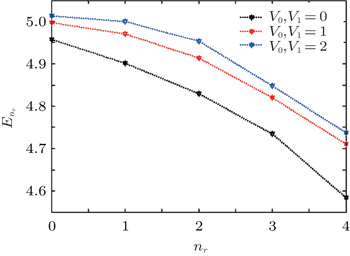Fig. 1. Energy eigenvalues for different values of V 0 and V 1 .

By varying parameters corresponding to values of δ and γ , some of the radial wavefunctions are listed in Table  3 . Radial wavefunctions for a particle under the influences of the Eckart potential and the trigonometric Manning Rosen potential are affected by potential constants V 0 and V 1 , and by parameter q as well. The parameter q has a dimension of inversed distance in space, which describes the effect range of the trigonometric Manning Rosen potential in space. If q is enlarged, it physically means that the potential range is smaller in a space. Table  3 and figure  2 show that the variation of n r for the radial wavefunction induces the distribution of particles to move away from or approach to the core of the atom. From Fig.  2 it follows that amplitude n r = 1 < n r = 2, which means that at n r = 2, the distribution of particles moves away from the nucleus compared with the case of smaller n r . This indicates that the higher the value of n r is, the greater the distance of the particle from the core is.Table 3.

Energy eigenvalues (in fm −1 ) with M = 5, C = 5, n l = 2, m = 2, v = 0.25, V 0 = 2, V 2 = 2, q = 0.2, α = 0.5, for a particle under the influences of the Eckart potential and the trigonometric Manning Rosen potential variation n r .

.
 Figure Option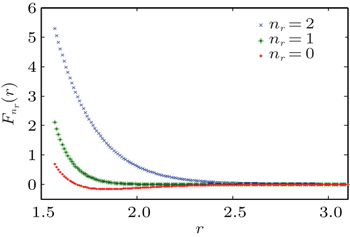Fig. 2. Radial wavefunction from Table  3 for different values of n r .

For the angular solution of the wavefunction in Eq. ( 54 ), we compare two spinor wavefunctions with the same angular quantum number n l = 4 but different values of α and β which corespond to parameters V 0 and V 1 in Fig.  3 . The plots are displayed in two and three dimensions together with the wave function. In Figs.  3(a) and 3(b) , α < β ; in Figs.  3(c) and 3(d) , α > β . For the same value of n l , the sum of waves does not change.

 Figure Option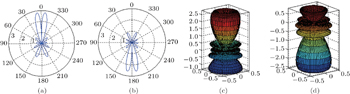Fig. 3. (a), (b) Two-dimensional and (c), (d) three-dimensional representations of the anglar wave function for a state n l = 4. In panels (a) and (b), Q ( θ ) = 1.57638(1.5865 + 16.793(−1 + cos( θ )) + 42.105(−1 + cos( θ )) 2 + 36.695(−1 + cos( θ )) 3 + 10.215(−1 + cos( θ )) 4 ) 2 (1−cos( θ )) 0.2 (1 + cos( θ )) 1.16 ; in panels (c) and (d), ( θ ) = 1.57638(6.7983 + 39.2793(−1 + cos( θ )) + 67.9901(−1 + cos( θ )) 2 + 45.3199(−1 + cos( θ )) 3 + 10.2495(−1 + cos( θ )) 4 ) 2 (1−cos( θ )) 1.4 (1 + cos( θ )) 0.27 .
 Figure Option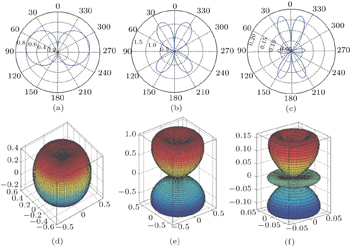Fig. 4. (a), (c) Polar diagrams and (d), (f) three-dimensional anguler wavefunctions with n l = 0 (a), (d), 1 (b), (e), and 2 (c), (f).
6. Conclusions

In this paper, we study the Dirac equation for particle spin-1/2 in the Eckart potential combined with the trigonometric Manning Rosen potential in condition that the scalar potential equals the vector potential. The radial part of the spinor wavefunction is obtained approximately from Eq. ( 44 ) and the angular part from Eq. ( 54 ). The results show that the disturbances of the Eckart and trigonometric Manning Rosen potentials change the radial part and the angular part of the wavefunction. The energy eigenvalue can be obtained via the asymptotic iteration method in Eq. ( 41 ) and the equation of orbital quantum number l as expressed in Eq. ( 52 ), where both are interrelated between quantum numbers. The energy spectrum is also numerically solved using Matlab software, showing that the increase of the radial quantum number n r causes the energy to decrease.

Reference
 1 Greiner W 2000 Relativistic Quantum Mechanics Berlin Springer 2 Hamzavi M Rajabi A A 2013 Hindawi Publishing Corporation Advances in High Energy Physics 2013 987632 3 Ginocchio J N Leviatan A Meng J Zhou S G 2004 Phys. Rev. C 69 3 4 Suparmi Cari 2014 ITB Journal Publisher 46 205 5 Suparmi A Cari C Angraini L M 2014 AIP Conf. Proc. 1615 111 6 Zhou S G Meng J Ring P 2003 Phys. Rev. Lett. 91 262501 7 Bahar M K Yasuk F 2012 Chin. Phys. B 22 010301 8 Hassanabadi H Ikot A N Zarrinkamar S 2014 Acta Phys. Polon. A 126 647 9 Bakkeshizadeh S Vahidi V 2012 Adv. Studies Theor. Phys. 6 733 10 Hassanabadi H Yazarloo B H Salehi N 2013 Indian J. Phys. 88 405 11 Aryanthy V D Suparmi C Saraswati I 2013 Seminar Nasional 2nd Lontar Physics Forum 2013 12 Ihtiari Saraswati I Suparmi Cari Seminar Nasional 2nd Lontar Physics Forum 2013 13 Meyur S Debnath S 2009 Lat. Am. J. Phys. Educ. 3 300 14 Suparmi A Cari C Deta U A 2014 Chin. Phys. B 23 090304 15 Kurniawan A Suparmi A Cari C 2015 Chin. Phys. B 24 030302 16 Sameer M I Babatunde J F Majid H 2013 Chin. Phys. Lett. 30 020305 17 Ikot A N Hassanabadi H Obong H P Umoren Y E C Isonguyo C N Yazarloo B H 2014 Chin. Phys. B 23 120303 18 Alsadi K S 2014 Chin. Phys. Lett. 31 120301 19 Soylu A Bayrak O Boztosun I 2008 J. Phys. A: Math. Theor. 41 065308 20 Falaye B J Hamzavi M Ikhdair S M 2012 arXiv:1207.1218v